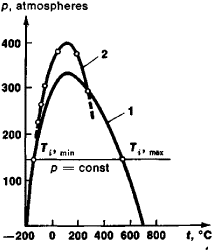# Inversion Curve

## Inversion Curve

a curve on a phase diagram that bounds the region of those phases (states) of a substance for which the transition of the substance from higher pressure to lower (in the process of throttling) is associated with a temperature drop. Beyond this region, the substance (a gas or liquid) heats on throttling. The constant-pressure line (isobar p = constant; see Figure 1) intersects the inversion curve at the two inversion points: the upper (Ti, max) and the lower (Ti, min). The upper point lies in the region of the gaseous state of the substance, and the lower in the liquid state.Figure 1. Inversion curves for air in pressure-temperature coordinates calculated according to (1) the Van der Waals equation and (2) experimental data

When Ti, min < T < Ti, max in a certain interval of pressure, the gas can be liquefied by throttling, but when T > Ti, max this cannot be accomplished. The closer a gas is in its properties to an ideal gas, the lower its inversion temperature (for hydrogen, for example, at atmospheric pressure Ti, max = −57°C, while for helium, Ti, max = −239°C). To liquefy helium by throttling, its temperature must first be reduced to −239°C.

IU. N. DROZHZHIN

Mentioned in ?
References in periodicals archive ?
But, it represents poorly the supercritical state: the properties of the fluids in this region are inaccurately predicted, in particular, derived properties such as the second virial coefficient [MATHEMATICAL EXPRESSION NOT REPRODUCIBLE IN ASCII] and the Joule-Thomson inversion curve.
A further comparison criteria for both attraction terms is the prediction of the Joule-Thomson inversion curve (JTIC).
However, even when the equations of state based on relations (4) and (7) don't describe the entire inversion curve accurately they exhibit a remarkable, surely not yet fulfilling, improvement of the JTIC as predicted by the SRK with Twu's attraction term.
When SRK1-b is compared to SRK1-a, we find a significant improvement in the supercritical region: a correct global behaviour, an accurate prediction of the second virial coefficient (Figure 9) on one side and a better description of the inversion curve (Figure 10) on the other side.
Matin, "A Comparison among Five Equations of State in Predicting the Inversion Curve of Some Fluids," Cryogenics.
As a result, the calculated high temperature branches of the inversion curves (Figure 14) are now more realistic.

Site: Follow: Share:
Open / Close